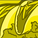# App Development

Discussion board where members can learn more about Qlik Sense App Development and Usage.

Announcements
QlikWorld Online 2021, May 10-12: Our Free, Virtual, Global Event REGISTER TODAY
cancel
Showing results for
Search instead for
Did you mean:Creator

## Set analysis with If statement creating a list to compare with

Hi Folks,

I try to do a set anaylsis, where I compare a dimension based upon the result of an If Statement to a list of values.

I tried different variations of =Only({<COL={\$(=If(\$(vLeftCol)=1,'1,3,5','2,4,6'))}>} COL), but didn't succeed.

In principle the Dimension COL can have values from 1 to 6 and if the variable vLeftCol = 1, then I Need the Statement to return 1, 3, 5 and otherwise it Needs to return 2, 4 and 6.

It would be great, if somebody had an idea how to solve this.

Best regards,

Dirk

Labels (3)

• ### Set Analysis

1 Solution

Accepted SolutionsCreator
Author

I found the solution.

Simply

=Only({<COL={\$(=If(\$(vLeftCol)=1,'1,3,5','2,4,6'))}>} COL)

is doing the trick.

Thank you very much for your help.

7 RepliesMVP

Where do you want to use this expression? KPI or chart expression or a calculated dimension? A bit of context would be helpful.Creator
Author

I want to use the set Analysis in a KPI (gauge) and I need to filter the correct columns. In order to make it easier understandable, I extracted the relevant part.Creator
Author

If  \$[vLeftCol)=1, then I want to get the results as shown in the table below 🤔

 COL Only({} COL 1 1 2 - 3 3 4 - 5 5 6 -MVP

With only this condition, you should use if statement, that would neither require set analysis nor only(). The way you are trying to write the set expression - seems would not work. If you could share a sample app with data and explain the complete expression scenario, we might help better.Creator
Author

Okay I try to explain it in a different way.

What I want to do is a set analysis, where I compare the Dimension COL depending on the value of the variable \$(vLeftCol).

So if \$(vLeftCol)=1, I want to have the set analysis doing COL={1,3,5) and otherwise I want to have the set Analysis checking for COL={2,4,6).

The full Expression is pretty lengthy, so I would prefer not to do it with an if statement enclosing it.

The Expression Only({<COL={\$(=If(\$(vLeftCol)=1,1,2))}>} COL), but I would like to have it working for 3 and 5 as well.Creator
Author

I found the solution.

Simply

=Only({<COL={\$(=If(\$(vLeftCol)=1,'1,3,5','2,4,6'))}>} COL)

is doing the trick.

Thank you very much for your help.MVP

Define another variable like:

vCondSet=If(\$(vLeftCol)=1, '1,3,5', '2,4,6'))

and then use this vCondSet in set analysis.